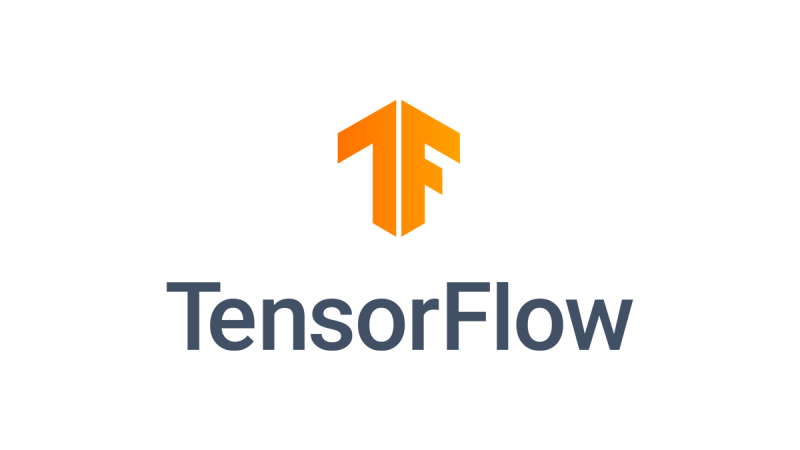# TensorFlow 2 教學：Keras-MNIST-VAE 變分自編碼器## Variational Autoencoder (VAE)

• 一般的 Autoencoder 將 input data 映射成 latent representation
• Varational Autoencoder 將 input data 映射到 distribution 的參數，像是高斯分佈的 mean 和 variance。

VAE 將不再只是產生一個無聊的、單一的向量來代表原來的圖片，而是產生一個 Gaussain Distribution，並且用他的平均值 (?) 及標準差 (?) 來代表。

1. 一張圖片會被 VAE 的 Encoder 映射到 Gaussian Distribution 的 ? 和 ?
2. 從以 ? 和 ? 代表的 Gaussian Distribution中抽取一個數值代表 latent variable
3. VAE 的 Decoder 再透過這個 latent variable 建構出原來的圖片

## Tensorflow2 實做 VAE

import tensorflow as tf
import numpy as np

mnist = tf.keras.datasets.mnist
(x_train, y_train), (x_test, y_test) = mnist.load_data()
# x_train.shape : (60000, 28, 28)
# x_test.shape  : (10000, 28, 28)

def preprocess_images(images):
images = images.reshape((images.shape, 28, 28, 1)) / 255.
images = np.where(images > .5, 1.0, 0.0).astype('float32')
return images

train_images = preprocess_images(x_train)
test_images = preprocess_images(x_test)
# train_images.shape : (60000, 28, 28, 1)
# test_images.shape  : (10000, 28, 28, 1)

tf.data.Dataset

train_size = train_images.shape # 60000
test_size  = test_images.shape #10000
batch_size = 32

train_dataset = (tf.data.Dataset.from_tensor_slices(train_images)
.shuffle(train_size).batch(batch_size))
test_dataset = (tf.data.Dataset.from_tensor_slices(test_images)
.shuffle(test_size).batch(batch_size))

tf.keras.Sequential

VAE 架構:

• Encoder : 將 input 圖片映射成 latent representation z
• input : x (圖片)
• output: Gaussian Distribution 的 ? 和 ? 。
• Decoder : 將 latent representation z 作為 input，output 出 image
• input : z (從 Gaussian Distribution 中 sample 值出來)
• output : x (圖片)

Reparameterization trick:

class VAE(tf.keras.Model):
# Variational Autoencdoer
# Encoder : 兩層 Convolutional layers
# Decoder : 三層 Convolutional layers
def __init__(self, latent_dim):
super(VAE, self).__init__()
self.latent_dim = latent_dim
self.encoder = tf.keras.Sequential(
[
tf.keras.layers.InputLayer(input_shape=(28, 28, 1)),
tf.keras.layers.Conv2D(
filters=32, kernel_size=3, strides=(2, 2), activation='relu'),
tf.keras.layers.Conv2D(
filters=64, kernel_size=3, strides=(2, 2), activation='relu'),
tf.keras.layers.Flatten(),
# No activation
tf.keras.layers.Dense(latent_dim + latent_dim),
]
)

self.decoder = tf.keras.Sequential(
[
tf.keras.layers.InputLayer(input_shape=(latent_dim,)),
tf.keras.layers.Dense(units=7*7*32, activation=tf.nn.relu),
tf.keras.layers.Reshape(target_shape=(7, 7, 32)),
tf.keras.layers.Conv2DTranspose(
activation='relu'),
tf.keras.layers.Conv2DTranspose(
activation='relu'),
# No activation
tf.keras.layers.Conv2DTranspose(
]
)

@tf.function
def sample(self, eps=None):
if eps is None:
eps = tf.random.normal(shape=(100, self.latent_dim))
return self.decode(eps, apply_sigmoid=True)

def encode(self, x):
mean, logvar = tf.split(self.encoder(x), num_or_size_splits=2, axis=1)
return mean, logvar

def reparameterize(self, mean, logvar):
eps = tf.random.normal(shape=mean.shape)
return eps * tf.exp(logvar * .5) + mean

def decode(self, z, apply_sigmoid=False):
logits = self.decoder(z)
if apply_sigmoid:
probs = tf.sigmoid(logits)
return probs
return logits

VAE 想做到的是最大化 ELBO(evidence lower bound)

## 參考資料Grandma